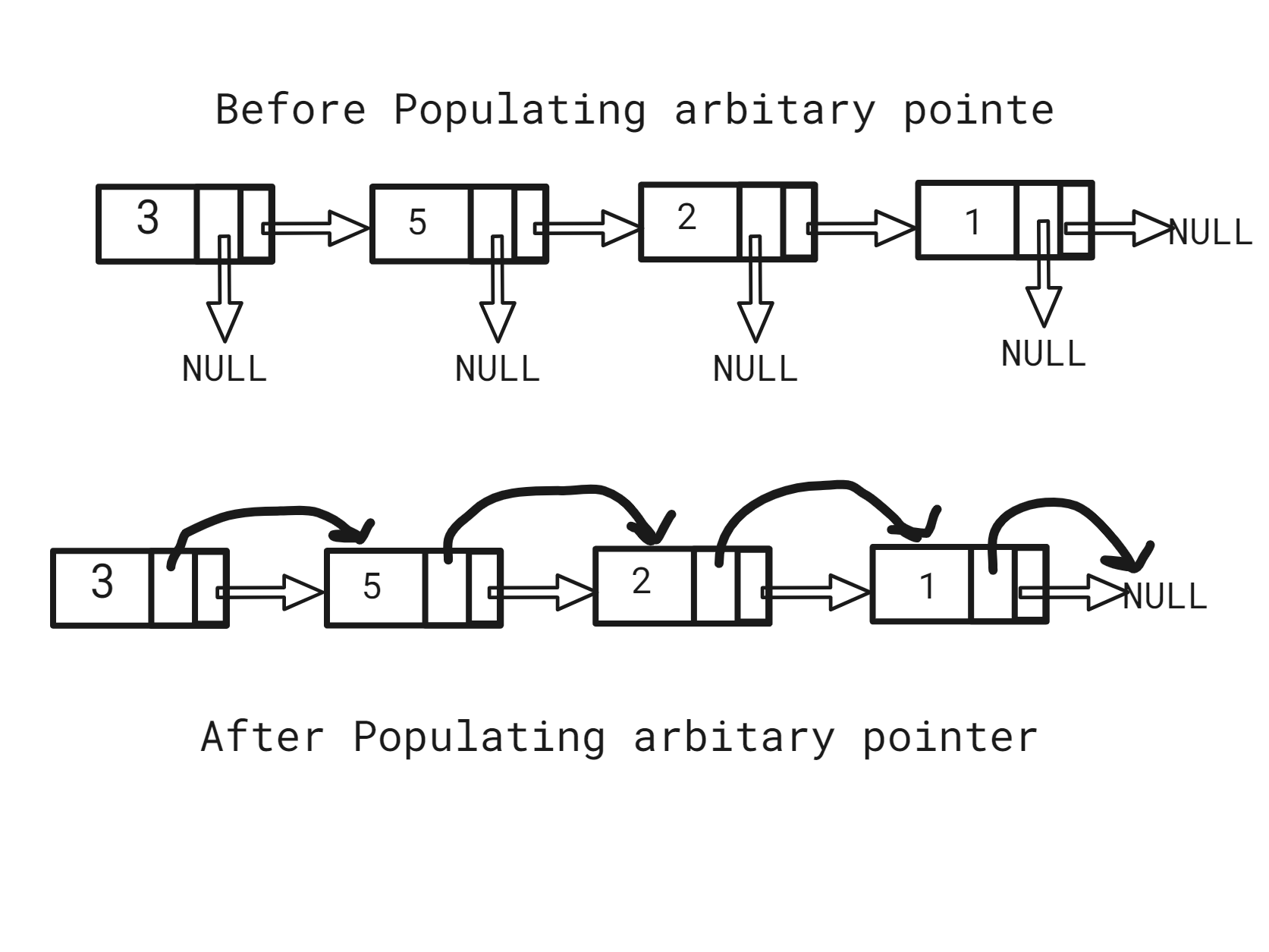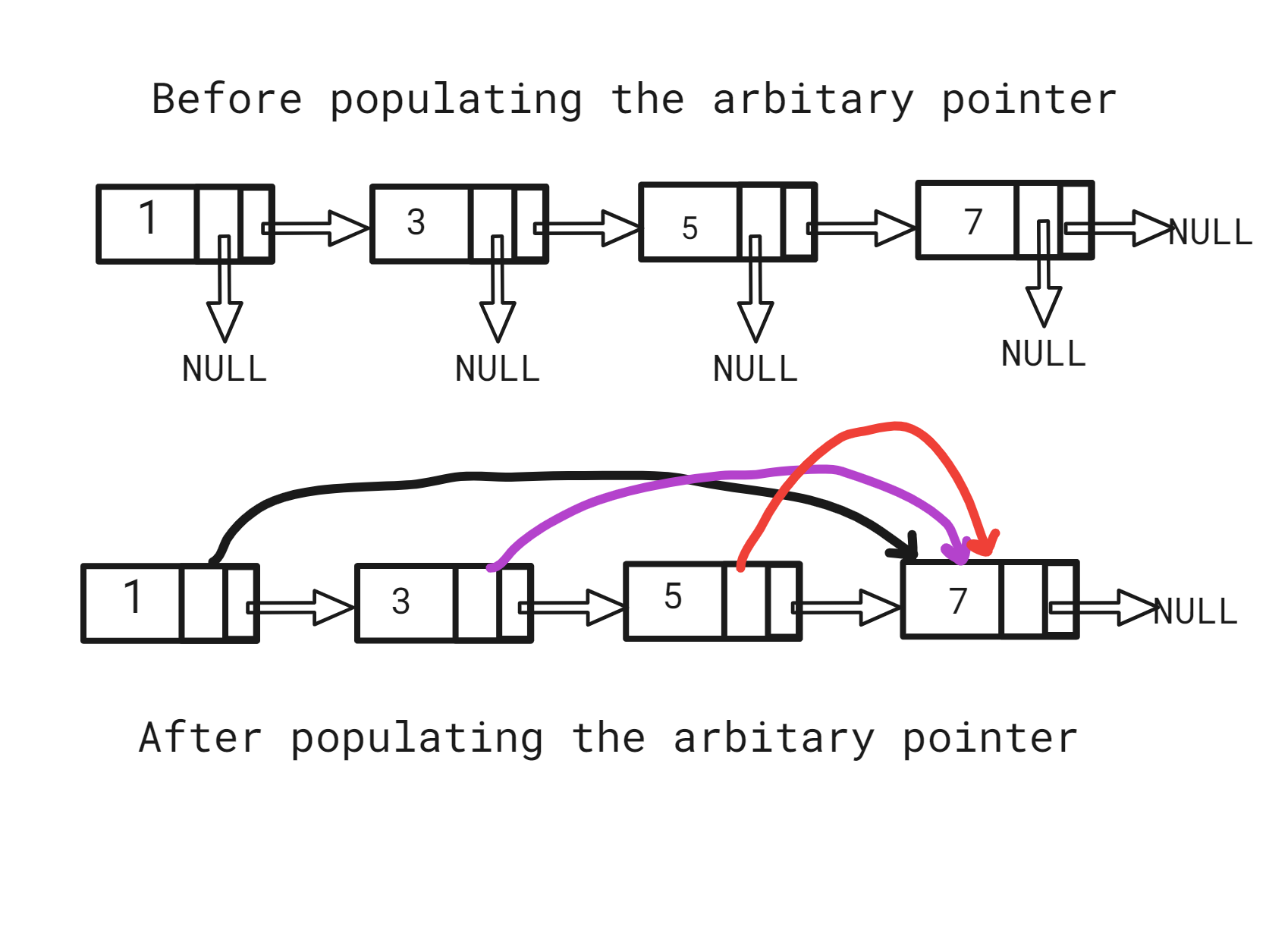New update is available. Click here to update.
Topics

# Point to Greatest Value Node

Easy0/40
Average time to solve is 10m## Problem statement

You are given a singly linked list with every node having an additional “arbitrary” pointer that currently points to NULL. We need to make the “arbitrary” pointer to the greatest value node in a linked list on its right side.

More formally, each Linked List node has three attributes ‘data’, which is the value of the node, the ‘next’ pointer, which points to the next node, and an ‘arbit’ pointer which is initially NULL.

Note:
``````You need to return the head after populating the ‘arbit’ pointer.
``````

For example:

``````Given ‘head’ as 3 -> 5 -> 2 -> 1.
````````````After populating the arbitrary pointer, each node's ‘arbit’ pointer would point to 5 2 1 -1 respectively.
``````
Detailed explanation ( Input/output format, Notes, Images )
Constraints:
``````1 <= ‘T’ <= 10
1 <= ‘N’ <= 5000
0 <= ‘data’ <= 10 ^ 4 and ‘data’ != -1

Time Limit: 1sec.
``````

#### Sample Input 1 :

``````2
3 5 2 1 -1
1 3 5 7 -1
``````

#### Sample Output 1 :

``````5 2 1 -1
7 7 7 -1
``````

#### Explanation of the Sample Input 1:

``````For the first test case :
````````````In the given Linked List, the above way will be how we populate the arbitrary pointer.

For the second test case:
````````````In the given Linked List, the above way will be how we populate the arbitrary pointer.
``````

#### Sample Input 2 :

``````2
4 3 2 1 -1
1 5 3 -1
``````

#### Sample Output 2 :

``````3 2 1 -1
5 3 -1
``````Console